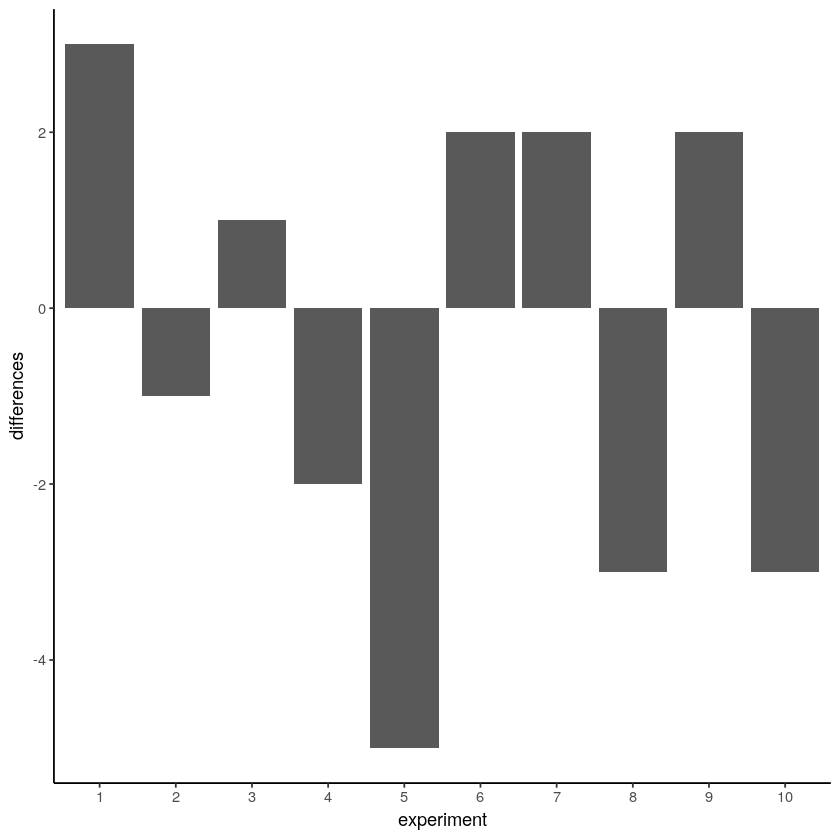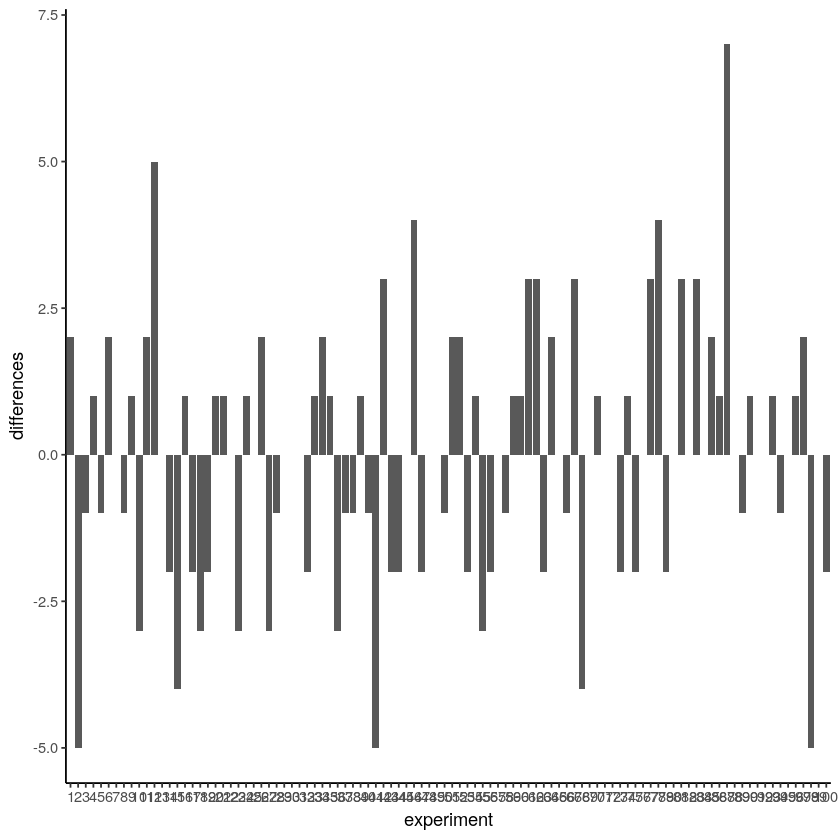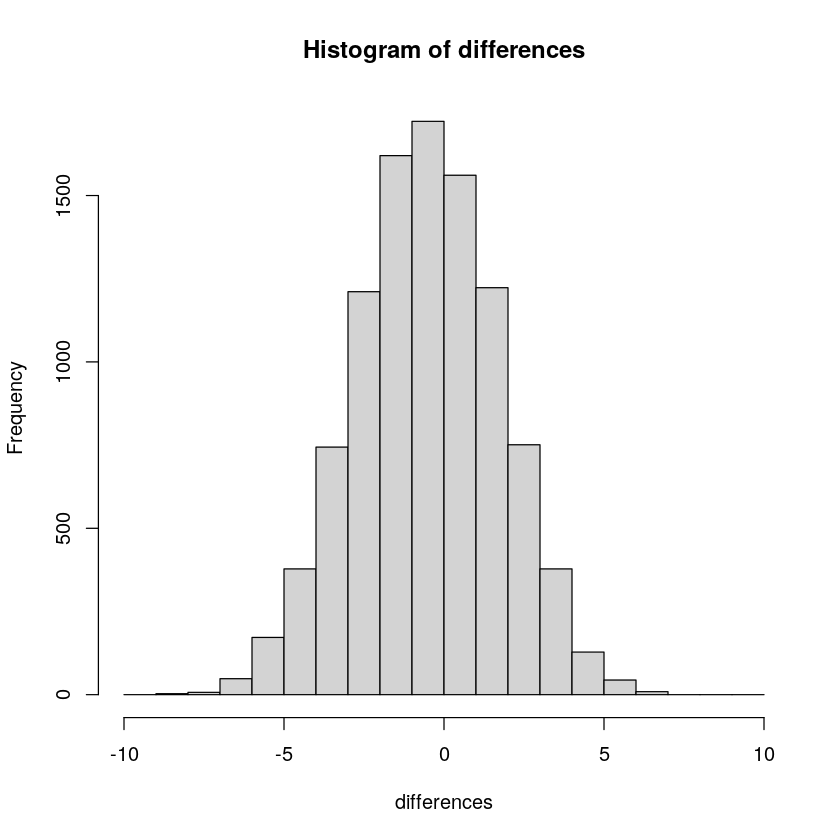# 5.4: Chance makes some differences more likely than others

$$\newcommand{\vecs}{\overset { \rightharpoonup} {\mathbf{#1}} }$$ $$\newcommand{\vecd}{\overset{-\!-\!\rightharpoonup}{\vphantom{a}\smash {#1}}}$$$$\newcommand{\id}{\mathrm{id}}$$ $$\newcommand{\Span}{\mathrm{span}}$$ $$\newcommand{\kernel}{\mathrm{null}\,}$$ $$\newcommand{\range}{\mathrm{range}\,}$$ $$\newcommand{\RealPart}{\mathrm{Re}}$$ $$\newcommand{\ImaginaryPart}{\mathrm{Im}}$$ $$\newcommand{\Argument}{\mathrm{Arg}}$$ $$\newcommand{\norm}{\| #1 \|}$$ $$\newcommand{\inner}{\langle #1, #2 \rangle}$$ $$\newcommand{\Span}{\mathrm{span}}$$ $$\newcommand{\id}{\mathrm{id}}$$ $$\newcommand{\Span}{\mathrm{span}}$$ $$\newcommand{\kernel}{\mathrm{null}\,}$$ $$\newcommand{\range}{\mathrm{range}\,}$$ $$\newcommand{\RealPart}{\mathrm{Re}}$$ $$\newcommand{\ImaginaryPart}{\mathrm{Im}}$$ $$\newcommand{\Argument}{\mathrm{Arg}}$$ $$\newcommand{\norm}{\| #1 \|}$$ $$\newcommand{\inner}{\langle #1, #2 \rangle}$$ $$\newcommand{\Span}{\mathrm{span}}$$$$\newcommand{\AA}{\unicode[.8,0]{x212B}}$$

OK, we have seen that chance can produce differences here. But, we still don’t have a good idea about what chance usually does and doesn’t do. For example, if we could find the window of opportunity here, we would be able find out that chance usually does not produce differences of a certain large size. If we knew what the size was, then if we ran experiment and our difference was bigger than what chance can do, we could be confident that chance did not produce our difference.

Let’s use the word difference some more, because it will be helpful. In fact, let’s think about our measure of green balls in terms of a difference. For example, in each experiment we counted the green balls for the left and right hand. What we really want to know is if there is a difference between them. So, we can calculate the difference score. Let’s decide that difference score = # of green gumballs in left hand - # of green gumballs in right hand. Now, we can redraw the 10 bar graphs from above. But this time we will only see one bar for each experiment. This bar will show the difference in number of green gumballs.Figure $$\PageIndex{1}$$: A look at the differences between number of each kind of gumball for the different replications. The difference should be zero, but sampling error produces non-zero differences.

Missing bars mean that there were an equal number of green gumballs chosen by the left and right hands (difference score is 0). A positive value means that more green gumballs were chosen by the left than right hand. A negative value means that more green gumballs were chosen by the right than left hand. Note that if we decided (and we get to decide) to calculate the difference in reverse (right hand - left hand), the signs of the differences scores would flip around.

We are starting to see more of the differences that chance can produce. The difference scores are mostly between -2 to +2. We could get an even better impression by running this pretend experiment 100 times instead of only 10 times. How about we do that.Figure $$\PageIndex{2}$$: Replicating the sampling 100 times, and looking at the differences each time. There are mnay kinds of differences that chance alone can produce.

Ooph, we just ran so many simulated experiments that the x-axis is unreadable, but it goes from 1 to 100. Each bar represents the difference of number of green balls chosen randomly by the left or right hand. Beginning to notice anything? Look at the y-axis, this shows the size of the difference. Yes, there are lots of bars of different sizes, this shows us that many kinds of differences do occur by chance. However, the y-axis is also restricted. It does not go from -10 to +10. Big differences greater than 5 or -5 don’t happen very often.

Now that we have a method for simulating differences due to chance, let’s run 10,000 simulated experiments. But, instead of plotting the differences in a bar graph for each experiment, how about we look at the histogram of difference scores. This will give us a clearer picture about which differences happen most often, and which ones do not. This will be another window into chance. The chance window of differences.Figure $$\PageIndex{3}$$: A histogram of the differences obtained by chance over 10,000 replications. The most frequency difference is 0, which is what we expect by chance. But the differences can be as large as -10 or +10. Larger differences occur less often by chance. Chance can’t do everything.

Our computer simulation allows us to force chance to operate hundreds of times, each time it produces a difference. We record the difference, then at the end of the simulation we plot the histogram of the differences. The histogram begins to show us the where the differences came from. Remember the idea that numbers come from a distribution, and the distribution says how often each number occurs. We are looking at one of these distributions. It is showing us that chance produces some differences more often than others. First, chance usually produces 0 differences, that’s the biggest bar in the middle. Chance also produces larger differences, but as the differences get larger (positive or negative), they occur less frequently. The shape of this histogram is your chance window, it tells you what chance can do, it tells you what chance usually does, and what it usually does not do.

You can use this chance window to help you make inferences. If you ran yourself in the gumball experiment and found that your left hand chose 2 more green gumballs than red gumballs, would you conclude that you left hand was special, and caused you to choose more green gumballs? Hopefully not. You could look at the chance window and see that differences of size +2 do happen fairly often by chance alone. You should not be surprised if you got a +2 difference. However, what if your left chose 5 more green gumballs than red gumballs. Well, chance doesn’t do this very often, you might think something is up with your left hand. If you got a whopping 9 more green gumballs than red gumballs, you might really start to wonder. This is the kind of thing that could happen (it’s possible), but virtually never happens by chance. When you get things that almost never happen by chance, you can be more confident that the difference reflects a causal force that is not chance.

This page titled 5.4: Chance makes some differences more likely than others is shared under a CC BY-SA 4.0 license and was authored, remixed, and/or curated by Matthew J. C. Crump via source content that was edited to the style and standards of the LibreTexts platform; a detailed edit history is available upon request.